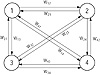# 24.C++ Neural Networks and Fuzzy Logic by Valluru B. Rao M&T Books, IDG Books Worldwide, Inc. ISBN: 1558515526   Pub Date: 06/01/95

## Chapter 4Constructing a Neural Network

### First Example for C++ Implementation

The neural network we presented in Chapter 1 is an example of a Hopfield network with a single layer. Now we present a C++ implementation of this network. Suppose we place four neurons, all connected to one another on this layer, as shown in Figure 4.1. Some of these connections have a positive weight and the rest have a negative weight. You may recall from the earlier presentation of this example, that we used two input patterns to determine the weight matrix. The network recalls them when the inputs are presented to the network, one at a time. These inputs are binary and orthogonal so that their stable recall is assured. Each component of a binary input pattern is either a 0 or a 1. Two vectors are orthogonal when their dot product—the sum of the products of their corresponding components—is zero. An example of a binary input pattern is 1 0 1 0 0. An example of a pair of orthogonal vectors is (0, 1, 0, 0, 1) and (1, 0, 0, 1, 0). An example of a pair of vectors that are not orthogonal is (0, 1, 0, 0, 1) and (1, 1, 0, 1, 0). These last two vectors have a dot product of 1, different from 0.Figure 4.1  Layout of a Hopfield Network

The two patterns we want the network to have stable recall for are A = (1, 0, 1, 0) and B = (0, 1, 0, 1). The weight matrix W is given as follows:

`                0    -3     3     -3      W     =  -3     0    -3      3                3    -3     0     -3               -3     3    -3      0 `

NOTE:  The positive links (values with positive signs) tend to encourage agreement in a stable configuration, whereas negative links (values with negative signs) tend to discourage agreement in a stable configuration.

We need a threshold function also, and we define it using a threshold value, [theta], as follows:

`                   1  if t >= [theta]      f(t) =  {                   0  if t < [theta] `

The threshold value [theta] is used as a cut-off value for the activation of a neuron to enable it to fire. The activation should equal or exceed the threshold value for the neuron to fire, meaning to have output 1. For our Hopfield network, [theta] is taken as 0. There are four neurons in the only layer in this network. The first node’s output is the output of the threshold function. The argument for the threshold function is the activation of the node. And the activation of the node is the dot product of the input vector and the first column of the weight matrix. So if the input vector is A, the dot product becomes 3, and f(3) = 1. And the dot products of the second, third, and fourth nodes become –6, 3, and –6, respectively. The corresponding outputs therefore are 0, 1, and 0. This means that the output of the network is the vector (1, 0, 1, 0), which is the same as the input pattern. Therefore, the network has recalled the pattern as presented. When B is presented, the dot product obtained at the first node is –6 and the output is 0. The activations of all the four nodes together with the threshold function give (0, 1, 0, 1) as output from the network, which means that the network recalled B as well. The weight matrix worked well with both input patterns, and we do not need to modify it.

#### Classes in C++ Implementation

In our C++ implementation of this network, there are the following classes: a network class, and a neuron class. In our implementation, we create the network with four neurons, and these four neurons are all connected to one another. A neuron is not self-connected, though. That is, there is no edge in the directed graph representing the network, where the edge is from one node to itself. But for simplicity, we could pretend that such a connection exists carrying a weight of 0, so that the weight matrix has 0’s in its principal diagonal.

The functions that determine the neuron activations and the network output are declared public. Therefore they are visible and accessible without restriction. The activations of the neurons are calculated with functions defined in the neuron class. When there are more than one layer in a neural network, the outputs of neurons in one layer become the inputs for neurons in the next layer. In order to facilitate passing the outputs from one layer as inputs to another layer, our C++ implementations compute the neuron outputs in the network class. For this reason the threshold function is made a member of the network class. We do this for the Hopfield network as well. To see if the network has achieved correct recall, you make comparisons between the presented pattern and the network output, component by component.C++ Neural Networks and Fuzzy Logic
ISBN: 1558515526
EAN: 2147483647
Year: 1995
Pages: 139

Similar book on Amazon